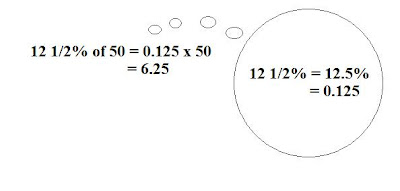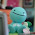## Sunday, January 16, 2011

### Final Percent Post

What is a percent? A percent is a way to show a number out of 100. You can use the sign '%' to show percent. Examples: 50%, it is also equal to 50/100 as a fraction or 0.5 as a decimal.

In our recent unit on percent, we learned how to represent percents in different ways, convert them into decimals and fractions, how to calculate the percent of a number, and how to combine percents.

Representing Percents:
- To represent a percent, you can shade squares on a grid of 100 squares called a hundred grid. One completely shaded grid represents 100%.100%

- To represent a percent greater than 100%, shade more than one grid.-To represent a fractional percent between 0% and 1%, shade part of one square.- To represent a fractional percent greater than 1%, shade squares from a hundred grid to show the whole number and part of one square from the grid to show the fraction.
Fractions, Decimals, Percents:
- Fractions, decimals, and percents can be used to represent numbers in various situations.

- Percents can be written as fractions and as decimals.Percent of a Number:

- You can use mental math strategies such as halving, doubling, and dividing by ten to find the percents of some numbers.

- To calculate the percent of a number, write the percent as a decimal and then multiply by the number.Combining Percents:
- Percents can be combined by adding to solve problems. 5% + 7% = 12% .

- To calculate the increase in a number,

• You can add the combined percent to the original number. 12% of 100 = 0.12 x 100 = 12
• You can multiply the original number by a single percent greater than 100. 112% of 100 = 1.12 x 100 = 112

- Percents of percents can be used to determine amounts that result from consecutive percent increases or decreases.

Here is my video about percents.

Here is a link to my percent scribe post:

http://spmath81610.blogspot.com/search/label/Olivia816

1.